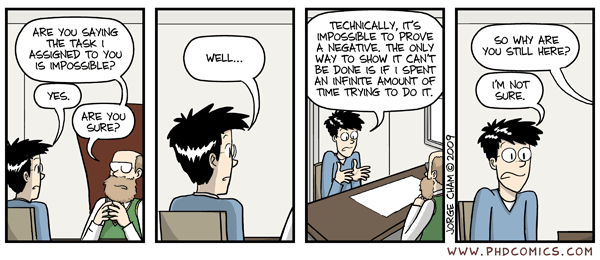# If we do not consider overfitting and regularization, should I try super hard to make training loss close to 0? Is there any lower bound for it?

Background: when build supervised machine learning models, the first step is usually to fit the training data well. Later on, we may add some regularizations to avoid overfitting.

My question is that, at the first step, should we try super hard to make the loss close to 0, by making the model super complex (say, millions of parameters or very deep neural network)? My experience is it seems there are some lower bound of the loss, that no matter what I do to intentionally overfit the training data, the loss would not decrease.

Why?

If the data is generated as $y_i = x_i+ \epsilon_i,\ \epsilon_i \sim\mathcal{N}(0, 1)$ then the best line of fit would not have zero error. In fact, no linear model could have zero error. This indicates that we shouldn't attempt to make the loss exactly zero (of course we would still like to make it small).

You can always make the loss zero by the following machine learning algorithm: $\text{fit}(D) = f$ where $f(x) = y$ iff $(x,y) \in D$ else $f(x) = 0$. Of course if you constrain yourself to more limited hypothesis spaces such as linear models or neural networks, the finite capacity of the model or optimization difficulties will prevent you from reaching exactly 0 error.

• +1, and for binary classification, even we can make 0-1 loss to 0 (do perfect job), the logistic loss will not be 0. Aug 7, 2018 at 8:20

The answer by @shimao covers the important points fairly well:

1. The "correct" model for your data will not be a model with zero training error. Thus, using zero training error as a criterion for model selection will not lead to the correct model.

2. Some non-parametric algorithms are able to memorize the training dataset theoretically (Neural networks, see this answer for more details) or practically (1-NN classifier). In such case, zero training error tells us nothing about the capability of the model to recover the underlying structure of the problem and model it well.

So, to sum up: No, don't try super hard to get zero training error. Training error is a useful indicator for debugging (and trying to overfit is a good debugging step), but you should not overdo it.

There is a very interesting old question along similar lines (although certainly not a duplicate), unfortunately missing a good conclusive answer. However, the thread links a comic describing the issue better than a long answer:• thanks for your answer +1. However, I would argue, if we have huge data. say billions of images, a good way to "memorize" training data is still a very valuable model. Aug 7, 2018 at 10:15